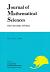## Vol. 21 (2014) No. 02Journal of Mathematical Sciences The University of Tokyo

1. Hoshi, Yuichiro
The Grothendieck Conjecture for Hyperbolic Polycurves of Lower Dimension
Vol. 21 (2014), No. 2, Page 153–219.

2. Sun, Zongliang
On Quadratic Differential Metrics on a Closed Riemann Surface
Vol. 21 (2014), No. 2, Page 221–234.

3. Liang, Song
A Mechanical Model of Brownian Motion with Uniform Motion Area
Vol. 21 (2014), No. 2, Page 235–334.
Honda Theory for Formal Groups of Abelian Varieties over $\mathbb Q$ of $\mathbf GL_{2}$-Type